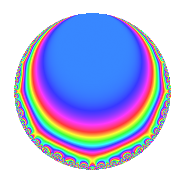Properties

 Label 8048.2.a.mLevel 8048 Weight 2 Character orbit 8048.a Self dual Yes Analytic conductor 64.264 Analytic rank 0 Dimension 3 CM No Inner twists 1

Related objects

Newspace parameters

 Level: $$N$$ = $$8048 = 2^{4} \cdot 503$$ Weight: $$k$$ = $$2$$ Character orbit: $$[\chi]$$ = 8048.a (trivial)

Newform invariants

 Self dual: Yes Analytic conductor: $$64.2636035467$$ Analytic rank: $$0$$ Dimension: $$3$$ Coefficient field: 3.3.257.1 Coefficient ring: $$\Z[a_1, a_2, a_3]$$ Coefficient ring index: $$1$$ Fricke sign: $$-1$$ Sato-Tate group: $\mathrm{SU}(2)$

$q$-expansion

Coefficients of the $$q$$-expansion are expressed in terms of a basis $$1,\beta_1,\beta_2$$ for the coefficient ring described below. We also show the integral $$q$$-expansion of the trace form.

 $$f(q)$$ $$=$$ $$q$$ $$-\beta_{1} q^{3}$$ $$+ ( -\beta_{1} + \beta_{2} ) q^{7}$$ $$+ \beta_{2} q^{9}$$ $$+O(q^{10})$$ $$q$$ $$-\beta_{1} q^{3}$$ $$+ ( -\beta_{1} + \beta_{2} ) q^{7}$$ $$+ \beta_{2} q^{9}$$ $$+ ( -4 + \beta_{1} ) q^{11}$$ $$+ ( 2 - \beta_{1} - \beta_{2} ) q^{13}$$ $$+ ( 4 + 2 \beta_{2} ) q^{17}$$ $$-4 q^{19}$$ $$+ ( 3 - \beta_{1} ) q^{21}$$ $$+ 4 q^{23}$$ $$-5 q^{25}$$ $$+ ( 2 \beta_{1} - \beta_{2} ) q^{27}$$ $$+ ( -2 \beta_{1} - 2 \beta_{2} ) q^{29}$$ $$+ ( 4 - 2 \beta_{1} + 2 \beta_{2} ) q^{31}$$ $$+ ( -3 + 4 \beta_{1} - \beta_{2} ) q^{33}$$ $$+ ( 2 - 4 \beta_{1} ) q^{37}$$ $$+ ( 3 - \beta_{1} + 2 \beta_{2} ) q^{39}$$ $$+ ( 2 \beta_{1} + 4 \beta_{2} ) q^{41}$$ $$+ ( -1 - 2 \beta_{1} + 3 \beta_{2} ) q^{43}$$ $$+ ( -7 + 2 \beta_{1} + \beta_{2} ) q^{47}$$ $$+ ( -1 - \beta_{1} - 2 \beta_{2} ) q^{49}$$ $$+ ( -6 \beta_{1} - 2 \beta_{2} ) q^{51}$$ $$+ ( 6 - 4 \beta_{1} - 2 \beta_{2} ) q^{53}$$ $$+ 4 \beta_{1} q^{57}$$ $$+ ( 1 + 2 \beta_{1} + \beta_{2} ) q^{59}$$ $$+ 2 q^{61}$$ $$+ ( 3 - 2 \beta_{2} ) q^{63}$$ $$+ ( -11 - 2 \beta_{1} + \beta_{2} ) q^{67}$$ $$-4 \beta_{1} q^{69}$$ $$+ ( -4 + 2 \beta_{2} ) q^{71}$$ $$+ ( 12 - \beta_{1} + 2 \beta_{2} ) q^{73}$$ $$+ 5 \beta_{1} q^{75}$$ $$+ ( -3 + 5 \beta_{1} - 4 \beta_{2} ) q^{77}$$ $$+ ( 4 - 5 \beta_{1} - 3 \beta_{2} ) q^{79}$$ $$+ ( -6 + \beta_{1} - 4 \beta_{2} ) q^{81}$$ $$+ ( \beta_{1} - \beta_{2} ) q^{83}$$ $$+ ( 6 + 2 \beta_{1} + 4 \beta_{2} ) q^{87}$$ $$+ ( 4 \beta_{1} + 2 \beta_{2} ) q^{89}$$ $$+ ( -3 \beta_{1} + 4 \beta_{2} ) q^{91}$$ $$+ ( 6 - 6 \beta_{1} ) q^{93}$$ $$+ ( -6 - 5 \beta_{1} + 3 \beta_{2} ) q^{97}$$ $$+ ( \beta_{1} - 3 \beta_{2} ) q^{99}$$ $$+O(q^{100})$$ $$\operatorname{Tr}(f)(q)$$ $$=$$ $$3q$$ $$\mathstrut -\mathstrut q^{3}$$ $$\mathstrut -\mathstrut q^{7}$$ $$\mathstrut +\mathstrut O(q^{10})$$ $$3q$$ $$\mathstrut -\mathstrut q^{3}$$ $$\mathstrut -\mathstrut q^{7}$$ $$\mathstrut -\mathstrut 11q^{11}$$ $$\mathstrut +\mathstrut 5q^{13}$$ $$\mathstrut +\mathstrut 12q^{17}$$ $$\mathstrut -\mathstrut 12q^{19}$$ $$\mathstrut +\mathstrut 8q^{21}$$ $$\mathstrut +\mathstrut 12q^{23}$$ $$\mathstrut -\mathstrut 15q^{25}$$ $$\mathstrut +\mathstrut 2q^{27}$$ $$\mathstrut -\mathstrut 2q^{29}$$ $$\mathstrut +\mathstrut 10q^{31}$$ $$\mathstrut -\mathstrut 5q^{33}$$ $$\mathstrut +\mathstrut 2q^{37}$$ $$\mathstrut +\mathstrut 8q^{39}$$ $$\mathstrut +\mathstrut 2q^{41}$$ $$\mathstrut -\mathstrut 5q^{43}$$ $$\mathstrut -\mathstrut 19q^{47}$$ $$\mathstrut -\mathstrut 4q^{49}$$ $$\mathstrut -\mathstrut 6q^{51}$$ $$\mathstrut +\mathstrut 14q^{53}$$ $$\mathstrut +\mathstrut 4q^{57}$$ $$\mathstrut +\mathstrut 5q^{59}$$ $$\mathstrut +\mathstrut 6q^{61}$$ $$\mathstrut +\mathstrut 9q^{63}$$ $$\mathstrut -\mathstrut 35q^{67}$$ $$\mathstrut -\mathstrut 4q^{69}$$ $$\mathstrut -\mathstrut 12q^{71}$$ $$\mathstrut +\mathstrut 35q^{73}$$ $$\mathstrut +\mathstrut 5q^{75}$$ $$\mathstrut -\mathstrut 4q^{77}$$ $$\mathstrut +\mathstrut 7q^{79}$$ $$\mathstrut -\mathstrut 17q^{81}$$ $$\mathstrut +\mathstrut q^{83}$$ $$\mathstrut +\mathstrut 20q^{87}$$ $$\mathstrut +\mathstrut 4q^{89}$$ $$\mathstrut -\mathstrut 3q^{91}$$ $$\mathstrut +\mathstrut 12q^{93}$$ $$\mathstrut -\mathstrut 23q^{97}$$ $$\mathstrut +\mathstrut q^{99}$$ $$\mathstrut +\mathstrut O(q^{100})$$

Basis of coefficient ring in terms of a root $$\nu$$ of $$x^{3}\mathstrut -\mathstrut$$ $$x^{2}\mathstrut -\mathstrut$$ $$4$$ $$x\mathstrut +\mathstrut$$ $$3$$:

 $$\beta_{0}$$ $$=$$ $$1$$ $$\beta_{1}$$ $$=$$ $$\nu$$ $$\beta_{2}$$ $$=$$ $$\nu^{2} - 3$$
 $$1$$ $$=$$ $$\beta_0$$ $$\nu$$ $$=$$ $$\beta_{1}$$ $$\nu^{2}$$ $$=$$ $$\beta_{2}\mathstrut +\mathstrut$$ $$3$$

Embeddings

For each embedding $$\iota_m$$ of the coefficient field, the values $$\iota_m(a_n)$$ are shown below.

For more information on an embedded modular form you can click on its label.

Label $$\iota_m(\nu)$$ $$a_{2}$$ $$a_{3}$$ $$a_{4}$$ $$a_{5}$$ $$a_{6}$$ $$a_{7}$$ $$a_{8}$$ $$a_{9}$$ $$a_{10}$$
1.1
 2.19869 0.713538 −1.91223
0 −2.19869 0 0 0 −0.364448 0 1.83424 0
1.2 0 −0.713538 0 0 0 −3.20440 0 −2.49086 0
1.3 0 1.91223 0 0 0 2.56885 0 0.656620 0
 $$n$$: e.g. 2-40 or 990-1000 Significant digits: Format: Complex embeddings Normalized embeddings Satake parameters Satake angles

Inner twists

This newform does not admit any (nontrivial) inner twists.

Atkin-Lehner signs

$$p$$ Sign
$$2$$ $$-1$$
$$503$$ $$1$$

Hecke kernels

This newform can be constructed as the intersection of the kernels of the following linear operators acting on $$S_{2}^{\mathrm{new}}(\Gamma_0(8048))$$:

 $$T_{3}^{3}$$ $$\mathstrut +\mathstrut T_{3}^{2}$$ $$\mathstrut -\mathstrut 4 T_{3}$$ $$\mathstrut -\mathstrut 3$$ $$T_{5}$$ $$T_{7}^{3}$$ $$\mathstrut +\mathstrut T_{7}^{2}$$ $$\mathstrut -\mathstrut 8 T_{7}$$ $$\mathstrut -\mathstrut 3$$ $$T_{13}^{3}$$ $$\mathstrut -\mathstrut 5 T_{13}^{2}$$ $$\mathstrut -\mathstrut 2 T_{13}$$ $$\mathstrut +\mathstrut 25$$